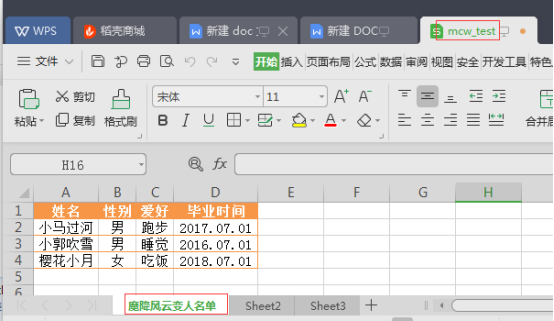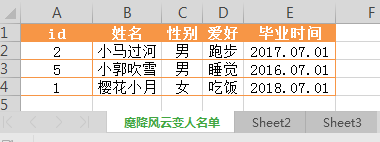xlrd模块学习1)# 打开Excel文件读取数据
import xlrd
workbook = xlrd.open_workbook('mcw_test.xlsx')
print(workbook)
----------------结果：
<xlrd.book.Book object at 0x02320410>
2) 打印所有的sheet列出所有的sheet名字
import xlrd
workbook = xlrd.open_workbook('mcw_test.xlsx')
print(workbook.sheet_names())
-----------------结果：
['魔降风云变人名单', 'Sheet2', 'Sheet3']
3）根据sheet索引或者名称获取sheet内容
import xlrd
workbook = xlrd.open_workbook('mcw_test.xlsx')
Data_sheet = workbook.sheets()
print(Data_sheet)
-----------------结果：
<xlrd.sheet.Sheet object at 0x02947730>
4）根据sheet索引或者名称获取sheet内容
import xlrd
workbook = xlrd.open_workbook('mcw_test.xlsx')
Data_sheet = workbook.sheet_by_name(u'魔降风云变人名单')
print(Data_sheet)
----------------结果：
<xlrd.sheet.Sheet object at 0x02947730>
5）获取sheet名称、行数和列数
import xlrd
workbook = xlrd.open_workbook('mcw_test.xlsx')
Data_sheet = workbook.sheet_by_name(u'魔降风云变人名单')
rows = Data_sheet.row_values(0) #获取第一行内容
cols = Data_sheet.col_values(1) #获取第二列内容
print(rows)
print(cols)
------------------------结果：
['姓名', '性别', '爱好', '毕业时间']
['性别', '', '', '']
6）
# 获取单元格内容的数据类型
# 相当于在一个二维矩阵中取值
# （row,col）-->(行,列)

import xlrd
workbook = xlrd.open_workbook('mcw_test.xlsx')
Data_sheet = workbook.sheet_by_name(u'魔降风云变人名单')
cell_A1 = Data_sheet.cell(0,0).value # 第一行第一列坐标A1的单元格数据
print(cell_A1)
---------------结果：

7)
import xlrd
workbook = xlrd.open_workbook('mcw_test.xlsx')
Data_sheet = workbook.sheet_by_name(u'魔降风云变人名单')
cell_C1 = Data_sheet.cell(0,2).value # 第一行第三列坐标C1的单元格数据
print(cell_C1)
--------------------结果：

8）
import xlrd
workbook = xlrd.open_workbook('mcw_test.xlsx')
Data_sheet = workbook.sheet_by_name(u'魔降风云变人名单')
rows = Data_sheet.row_values(0) #获取第一行内容
cols = Data_sheet.col_values(1) #获取第二列内容
cell_B1 = Data_sheet.row(0).value # 第1行第2列
cell_D2 = Data_sheet.col(3).value # 第4列第2行
print(cell_B1)
print(cell_D2)
-----------------结果：

2017.07.01
9# 检查单元格的数据类型
# ctype的取值含义
# ctype : 0 empty,1 string, 2 number, 3 date, 4 boolean, 5 error
import xlrd
workbook = xlrd.open_workbook('mcw_test.xlsx')
Data_sheet = workbook.sheet_by_name(u'魔降风云变人名单')
print(Data_sheet.cell(3,0).ctype)
print(Data_sheet.cell(1,3).ctype)
--------------结果：
1
1
10# 读取excel中单元格内容为日期的方式
date_value = xlrd.xldate_as_tuple(Data_sheet.cell_value(4,0),workbook.datemode)

print(date_value) # -->(2017, 9, 6, 0, 0, 0)

print('%d:%d:%d' %(date_value[3:])) # 打印时间
print('%d/%02d/%02d' %(date_value[0:3])) # 打印日期

第一个工作表的名字，总行数nrows，总列数

import xlrd
workbook = xlrd.open_workbook('mcw_test.xlsx')
Data_sheet = workbook.sheets()   #索引为0的工作表sheet，即第一个工作表
print(Data_sheet.name,Data_sheet.nrows,Data_sheet.ncols)  #第一个工作表的名字，总行数nrows，总列数

--------------结果；

如下表，循环获取表格所有行:

（#rows获取到表格行数，从0到表格行数做循环。利用索引取指定索引的行的值）

import xlrd
workbook = xlrd.open_workbook('mcw_test.xlsx')
Data_sheet = workbook.sheets()   #索引为0的工作表sheet，即第一个工作表
rows=Data_sheet.nrows
for i in range(0,rows):
print(Data_sheet.row_values(i),type(Data_sheet.row_values(i)))
------------------结果：
['id', '姓名', '性别', '爱好', '毕业时间'] <class 'list'>
[2.0, '小马过河', '', '跑步', '2017.07.01'] <class 'list'>
[5.0, '小郭吹雪', '', '睡觉', '2016.07.01'] <class 'list'>
[1.0, '樱花小月', '', '吃饭', '2018.07.01'] <class 'list'>

#rows获取到表格行数，从0到表格行数做循环。利用索引取指定索引的行的值获取表格所有列内容：

import xlrd
workbook = xlrd.open_workbook('mcw_test.xlsx')
Data_sheet = workbook.sheets()   #索引为0的工作表sheet，即第一个工作表
cols=Data_sheet.ncols #第一个工作表的名字，总行数nrows，总列数
for j in range(0,cols):
print(Data_sheet.col_values(j))
---------------结果：
['id', 2.0, 5.0, 1.0]
['姓名', '小马过河', '小郭吹雪', '樱花小月']
['性别', '', '', '']
['爱好', '跑步', '睡觉', '吃饭']
['毕业时间', '2017.07.01', '2016.07.01', '2018.07.01']

#获取小郭吹雪（未知行）的所有信息（一个人一行信息），以及单独拿出毕业时间（可以查看出已知列）import xlrd
workbook = xlrd.open_workbook('mcw_test.xlsx')
Data_sheet = workbook.sheets()   #索引为0的工作表sheet，即第一个工作表“魔降风云变人名单”
name=Data_sheet.col_values(1)  # 工作表.col_values(1)是取表格第二列“姓名”列。name单元格为元素形成的列表，第一个值为“姓名”，索引行为0
for i in range(0,Data_sheet.nrows):  # Data_sheet.nrows是工作表.nrows，即这张工作表的总行数。
if name[i]=="小郭吹雪":  #name为第二列的列表，在列表中找到索引行为i且列表元素为"小郭吹雪"的值，如果找到，就有了行数
print("%s的索引行号是:%s，也是表格第(%s)行内容"%(name[i],i,i+1)) #循环后得到"小郭吹雪"的索引行，打印出“姓名”列的元素即“小郭吹雪”
print("小郭吹雪的毕业时间是:%s"%Data_sheet.row(i).value) #工作表.行（行索引）[列索引].value获得“小郭吹雪”的毕业时间。毕业时间索引是看表结构已知的
break  #找到“小郭吹雪”后，就停止循环。节省计算资源
----------------------------结果：

#综上：想要找到表格某列的一个元素，求这个元素的本行其它内容：找到表格总行数，对本列的元素循环，可以找到这个元素所在的行数。这样就可以用代码求得本行其它列的内容了。

import xlrd
workbook = xlrd.open_workbook('mcw_test.xlsx')
Data_sheet = workbook.sheets()
name=Data_sheet.col_values(1)
for i in range(0,Data_sheet.nrows):
if name[i]=="小郭吹雪":
print("%s的索引行号是:%s，也是表格第(%s)行内容"%(name[i],i,i+1))
print("小郭吹雪的毕业时间是:%s"%Data_sheet.row(i).value)
break

1）https://blog.csdn.net/qq_41185868/article/details/80469355

posted @ 2019-04-19 16:05  马昌伟  阅读(5579)  评论(0编辑  收藏  举报